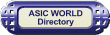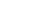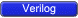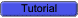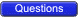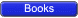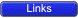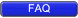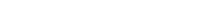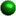Introduction

Verilog has built in primitives like gates, transmission gates, and switches. These are rarely used in design (RTL Coding), but are used in post synthesis world for modeling the ASIC/FPGA cells; these cells are then used for gate level simulation, or what is called as SDF simulation. Also the output netlist format from the synthesis tool, which is imported into the place and route tool, is also in Verilog gate level primitives.Note : RTL engineers still may use gate level primitivies or ASIC library cells in RTL when using IO CELLS, Cross domain synch cells.Gate Primitives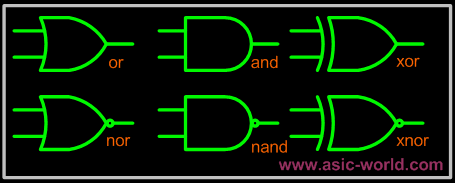The gates have one scalar output and multiple scalar inputs. The 1st terminal in the list of gate terminals is an output and the other terminals are inputs.Gate Description and N-input AND gate nand N-input NAND gate or N-input OR gate nor N-input NOR gate xor N-input XOR gate xnor N-input XNOR gateExamples```
1 module gates();
2
3 wire out0;
4 wire out1;
5 wire out2;
6 reg  in1,in2,in3,in4;
7
8 not U1(out0,in1);
9 and U2(out1,in1,in2,in3,in4);
10 xor U3(out2,in1,in2,in3);
11
12 initial begin
13   \$monitor(
14   "in1=%b in2=%b in3=%b in4=%b out0=%b out1=%b out2=%b",
15    in1,in2,in3,in4,out0,out1,out2);
16   in1 = 0;
17   in2 = 0;
18   in3 = 0;
19   in4 = 0;
20    #1  in1 = 1;
21    #1  in2 = 1;
22    #1  in3 = 1;
23    #1  in4 = 1;
24    #1  \$finish;
25 end
26
27 endmodule
`````` in1 = 0 in2 = 0 in3 = 0 in4 = 0 out0 = 1 out1 = 0 out2 = 0
in1 = 1 in2 = 0 in3 = 0 in4 = 0 out0 = 0 out1 = 0 out2 = 1
in1 = 1 in2 = 1 in3 = 0 in4 = 0 out0 = 0 out1 = 0 out2 = 0
in1 = 1 in2 = 1 in3 = 1 in4 = 0 out0 = 0 out1 = 0 out2 = 1
in1 = 1 in2 = 1 in3 = 1 in4 = 1 out0 = 0 out1 = 1 out2 = 1
```Transmission Gate Primitives

Transmission gates are bi-directional and can be resistive or non-resistive.Syntax: keyword unique_name (inout1, inout2, control);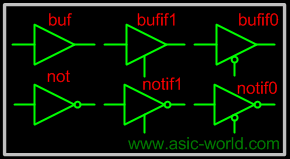Gate Description not N-output inverter buf N-output buffer. bufif0 Tri-state buffer, Active low en. bufif1 Tri-state buffer, Active high en. notif0 Tristate inverter, Low en. notif1 Tristate inverter, High en.Transmission gates tran and rtran are permanently on and do not have a control line. Tran can be used to interface two wires with seperate drives, and rtran can be used to weaken signals.Examples```
1 module transmission_gates();
2
3 reg data_enable_low, in;
4 wire data_bus, out1, out2;
5
6 bufif0 U1(data_bus,in, data_enable_low);
7 buf  U2(out1,in);
8 not U3(out2,in);
9
10 initial begin
11   \$monitor(
12     "@%g in=%b data_enable_low=%b out1=%b out2= b data_bus=%b",
13     \$time, in, data_enable_low, out1, out2, data_bus);
14   data_enable_low = 0;
15   in = 0;
16    #4  data_enable_low = 1;
17    #8  \$finish;
18 end
19
20 always  #2  in = ~in;
21
22 endmodule
`````` @0 in = 0 data_enable_low = 0 out1 = 0 out2 = 1 data_bus = 0
@2 in = 1 data_enable_low = 0 out1 = 1 out2 = 0 data_bus = 1
@4 in = 0 data_enable_low = 1 out1 = 0 out2 = 1 data_bus = z
@6 in = 1 data_enable_low = 1 out1 = 1 out2 = 0 data_bus = z
@8 in = 0 data_enable_low = 1 out1 = 0 out2 = 1 data_bus = z
@10 in = 1 data_enable_low = 1 out1 = 1 out2 = 0 data_bus = z
```Switch Primitives

There are six different switch primitives (transistor models) used in Verilog, nmos, pmos and cmos and the corresponding three resistive versions rnmos, rpmos and rcmos. The cmos type of switches have two gates and so have two control signals.Syntax: keyword unique_name (drain. source, gate)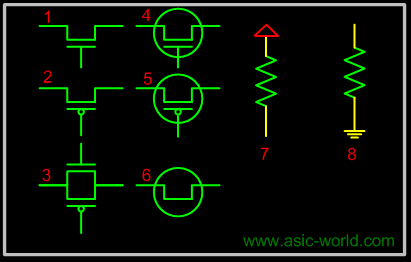Gate Description 1. pmos Uni-directional PMOS switch 1. rpmos Resistive PMOS switch 2. nmos Uni-directional NMOS switch 2. rnmos Resistive NMOS switch 3. cmos Uni-directional CMOS switch 3. rcmos Resistive CMOS switch 4. tranif1 Bi-directional transistor (High) 4. tranif0 Bi-directional transistor (Low) 5. rtranif1 Resistive Transistor (High) 5. rtranif0 Resistive Transistor (Low) 6. tran Bi-directional pass transistor 6. rtran Resistive pass transistor 7. pullup Pull up resistor 8. pulldown Pull down resistorTransmission gates are bi-directional and can be resistive or non-resistive. Resistive devices reduce the signal strength which appears on the output by one level. All the switches only pass signals from source to drain, incorrect wiring of the devices will result in high impedance outputs.Examples```
1 module switch_primitives();
2
3 wire  net1, net2, net3;
4 wire  net4, net5, net6;
5
6 tranif0 my_gate1 (net1, net2, net3);
7 rtranif1 my_gate2 (net4, net5, net6);
8
9 endmodule
```Transmission gates tran and rtran are permanently on and do not have a control line. Tran can be used to interface two wires with separate drives, and rtran can be used to weaken signals. Resistive devices reduce the signal strength which appears on the output by one level. All the switches only pass signals from source to drain, incorrect wiring of the devices will result in high impedance outputs.Logic Values and signal Strengths

The Verilog HDL has got four logic valuesLogic Value Description 0 zero, low, false 1 one, high, true z or Z high impedance, floating x or X unknown, uninitialized, contentionVerilog Strength LevelsStrength Level Specification Keyword 7 Supply Drive supply0 supply1 6 Strong Pull strong0 strong1 5 Pull Drive pull0 pull1 4 Large Capacitance large 3 Weak Drive weak0 weak1 2 Medium Capacitance medium 1 Small Capacitance small 0 Hi Impedance highz0 highz1Example : Strength Level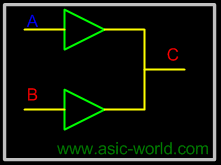Two buffers that has output

A : Pull 1

B : Supply 0

Since supply 0 is stronger then pull 1, Output C takes value of B.Example 2 : Strength Level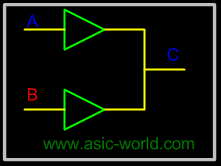Two buffers that has output

A : Supply 1

B : Large 1

Since Supply 1 is stronger then Large 1, Output C takes the value of A